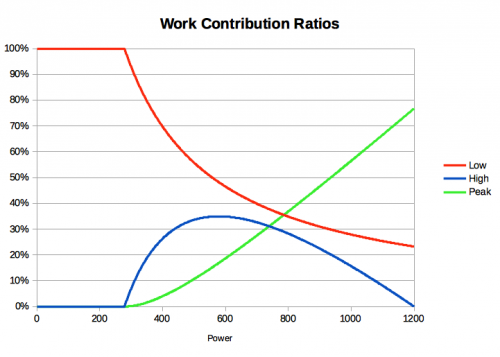Sometimes referred to as Work Contribution Ratios, these ratios divide work and strain into peak, high and low.  Since the three Fitness Signature parameters can be used to determine and predict your maximal power available, an athlete’s ability to perform must work integratively into each of these parameters.  That is, each parameter represents an integrated fitness measure and all three together can be used to effectively represent an athlete’s fitness.  This is true because MPA, which is derived from the Fitness Signature, is predictive of an athlete’s ability to perform.

Therefore, we can assign for each joule of work performed, how much influence each fitness signature parameter had in the production of the work.  Similarly, we can assign for each joule of strain, how much influence each fitness signature parameter had in the generation of that strain.  When we do so, we allocate work or strain according to these ratios.

• Low Work / Strain / Training Load / Recovery Load / XSS is determined by Threshold Power
• High Work / Strain / Training Load / Recovery Load / XSS is determined by High Intensity Energy
• Peak Work / Strain / Training Load / Recovery Load / XSS is determined by Peak Power